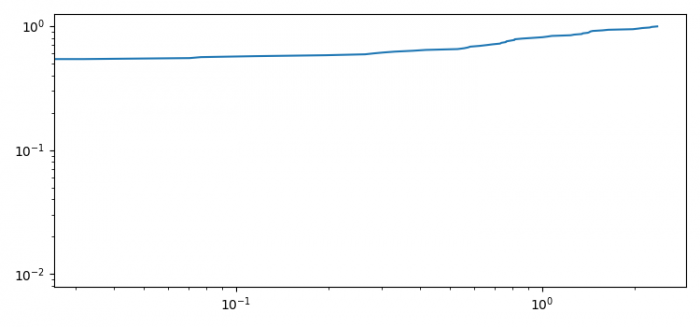# How to show the Logarithmic plot of a cumulative distribution function in Matplotlib?

To show the Logarithmic plot of a cumulative distribution function in Matplotlib, we can take the following steps.

## Steps

• Set the figure size and adjust the padding between and around the subplots.
• Initialize a variable, N, for number of sample data.
• Create data, X2 and F2 using numpy.
• Plot X2 and F2 using plot() method.
• Make x and y scale logarithmic.
• To display the figure, use show() method.

## Example

import numpy as np
import matplotlib.pyplot as plt

plt.rcParams["figure.figsize"] = [7.50, 3.50]
plt.rcParams["figure.autolayout"] = True

N = 100
data = np.random.randn(N)
X2 = np.sort(data)
F2 = np.array(range(N))/float(N)

plt.plot(X2, F2)

plt.xscale('log')
plt.yscale('log')

plt.show()

## Output